How to Learn in 24 Hours?

 Need Help? M-F: 9am-5pm(PST): Toll-Free: (877) RAPID-10 or 1-877-727-4310 24/7 Online Technical Support: The Rapid Support Center vip@rapidlearningcenter.com Secure Online Order:Need Proof? Testimonials by Our Users

 Rapid Learning Courses: MCAT in 24 Hours (2021-22) USMLE in 24 Hours (Boards) Chemistry in 24 Hours Biology in 24 Hours Physics in 24 Hours Mathematics in 24 Hours Psychology in 24 Hours SAT in 24 Hours ACT in 24 Hours AP in 24 Hours CLEP in 24 Hours DAT in 24 Hours (Dental) OAT in 24 Hours (Optometry) PCAT in 24 Hours (Pharmacy) Nursing Entrance Exams Certification in 24 Hours eBook - Survival Kits Audiobooks (MP3)

 Tell-A-Friend: Have friends taking science and math courses too? Tell them about our rapid learning system.Home »  Mathematics »  Pre-Calculus

Vector and Complex Numbers

 Topic Review on "Title": The Magnitude of a vector: Let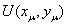be a vector then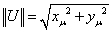. The dot product of vectors: Letand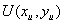be two vectors then the dot product of V and U is equal to: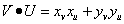. Definition of a complex number: A complex number is a number of the standard form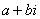where a and b are real numbers and. The complex conjugate: The conjugate of a complex numberis a complex number equal to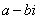. The complex plane: The two-dimensional Cartesian coordinate system where a complex number is viewed as a point. DeMoivre’s Formula: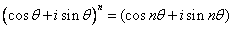where n is an integer. Exponential form of complex number: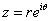where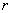is the modulus of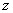and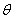is its argument.

Rapid Study Kit for "Title":
 Flash Movie Flash Game Flash Card Core Concept Tutorial Problem Solving Drill Review Cheat Sheet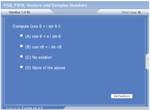"Title" Tutorial Summary : Vectors are common quantities that are used in mathematics and in other field of studies. The properties of vectors are discussed in the examples and their use in application problems. The graphical representation of vectors allows for the introduction of the magnitude of a vector. Complex numbers are introduced in this part of tutorial along with their properties such as the addition, subtraction, multiplication and division of complex numbers. Other representations of complex numbers are presented such as the trigonometric and the exponential ones. Famous formulas such as De Moivre’s Formulas are used in examples to calculate complex numbers.

 Tutorial Features: Specific Tutorial Features: • Graphical illustration of the operations of vectors and the formulation of complex numbers. • Diagrams showing the features of vectors. • Graphical representation of complex numbers. • The use of true or false questions to verify understanding of the complex number properties. Series Features: • Concept map showing inter-connections of new concepts in this tutorial and those previously introduced. • Definition slides introduce terms as they are needed. • Visual representation of concepts • Animated examples—worked out step by step • A concise summary is given at the conclusion of the tutorial.

 "Title" Topic List: Definition of a vector Magnitude of a vector Addition of vectors Opposite vectors Vector dot productComplex Numbers Definition of complex numbers The imaginary unit The complex conjugate Addition of complex numbers Subtraction of complex numbers Multiplying complex numbers Multiplying a complex number and its conjugate Dividing complex numbers Trigonometric formulation of complex numbers DeMoivre’s Formula Exponential form of a complex number

See all 24 lessons in Pre-Calculus, including concept tutorials, problem drills and cheat sheets:
Teach Yourself Pre-Calculus Visually in 24 Hours